# ISEE Middle Level Quantitative : How to find the area of a square

## Example Questions

### Example Question #1 : Squares

Calvin is remodeling his room.  He usedfeet of molding to put molding around all four walls.  Now he wants to paint three of the walls.  Each wall is the same width and isfeet tall. If one can of paint coverssquare feet, how many cans of paint will he need to paint three walls.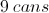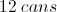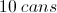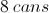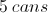Explanation:

When Calvin put upfeet of molding, he figured out the perimeter of the room wasfeet.  Since he knows that all four walls are the same width, he can use the equation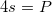to determine the length of each side by pluggingin forand solving for.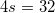In order to solve for, Calvin must divide both sides by four.

The left-hand side simplifies to: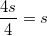The right-hand side simplifies to: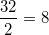Now, Calvin knows the width of each room isfeet.  Next he must find the area of each wall. To do this, he must multiply the width by the height because the area of a rectangle is found using the equation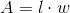. Since Calvin now knows that the width of each wall isfeet and that the height of each wall is alsofeet, he can multiply the two together to find the area.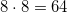Since Calvin wants to find how much paint he needs to cover three walls, he must first find out how many square feet he is covering.  If one wall issquare feet, he must multiply that by.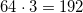Calvin is painting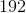square feet. If one can of paint covers 24 square feet, he must divide the total space (square feet) by.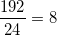Calvin will needcans of paint.

### Example Question #1 : Squares

Which is the greater quantity?

(a) The surface area of a cube with volume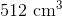(b) The surface area of a cube with sidelength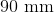(b) is greater

(a) is greater

(a) and (b) are equal

It is impossible to tell from the information given

(b) is greater

Explanation:

We can actually solve this by comparing volumes; the cube with the greater volume has the greater sidelength and, subsequently, the greater surface area.

The volume of the cube in (b) is the cube of 90 millimeters, or 9 centimeters. This is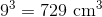, which is greater than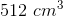. The cube in (b) has the greater volume, sidelength, and, most importantly, surface area.

### Example Question #401 : Isee Middle Level (Grades 7 8) Quantitative Reasoning

The sum of the lengths of three sides of a square is one yard. Give its area in square inches.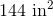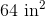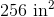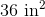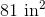Explanation:

A square has four sides of the same length.

One yard is equal to 36 inches, so each side of the square has length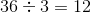inches.

Its area is the square of the sidelength, or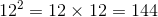square inches.

### Example Question #11 : Geometry

The sum of the lengths of three sides of a square is 3,900 centimeters. Give its area in square meters.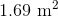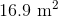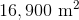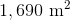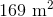Explanation:

100 centimeters are equal to one meter, so 3,900 centimeters are equal to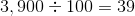meters.

A square has four sides of the same length. Since the sum of the lengths of three of the congruent sides is 3,900 centimeters, or 39 meters, each side measures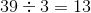meters.

The area of the square is the square of the sidelength, or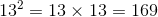square meters.

### Example Question #1 : Plane Geometry

Each side of a square is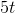units long. Which is the greater quantity?

(A) The area of the square

(B)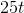It is impossible to determine which is greater from the information given

(B) is greater

(A) and (B) are equal

(A) is greater

It is impossible to determine which is greater from the information given

Explanation:

The area of a square is the square of its side length: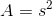Using the side length from the question: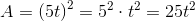However, it is impossible to tell with certainty which of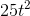andis greater.

For example, if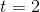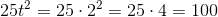and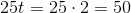so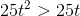if.

But if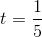,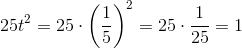and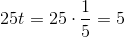so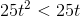if.

### Example Question #2 : How To Find The Area Of A Square

A square has a side with a length of 5. What is the area of the square?Explanation:

The area formula for a square is length times width. Keep in mind that all of a square's sides are equal.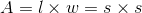So, if one side of a square equals 5, all of the other sides must also equal 5. You will find the area of the square by multiplying two of its sides: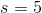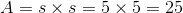### Example Question #1 : Plane Geometry

One square mile is equivalent to 640 acres. Which of the following is the greater quantity?

(a) The area of a square plot of land whose perimeter measures one mile

(b) 160 acres

(a) is the greater quantity

(a) and (b) are equal

It is impossible to determine which is greater from the information given

(b) is the greater quantity

(b) is the greater quantity

Explanation:

A square plot of land with perimeter one mile has as its sidelength one fourth of this, ormile; its area is the square of this, or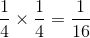square miles.

One square mile is equivalent to 640 acres, so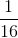square miles is equivalent to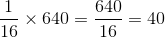acres.

This makes (b) greater.

### Example Question #1 : Plane Geometry

One square kilometer is equal to 100 hectares.

Which is the greater quantity?

(a) The area of a rectangular plot of land 500 meters in length and 200 meters in width

(b) One hectare

It is impossible to determine which is greater from the information given

(a) and (b) are equal

(a) is the greater quantity

(b) is the greater quantity

(a) is the greater quantity

Explanation:

One kilometer is equal to 1,000 meters, so divide each dimension of the plot in meters by 1,000 to convert to kilometers: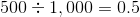kilometers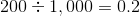kilometers

Multiply the dimensions to get the area in square kilometers: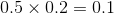square kilometers

Since one square kilometer is equal to 100 hectares, multiply this by 100 to convert to hectares: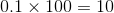hectares

This makes (a) the greater.

### All ISEE Middle Level Quantitative Resources